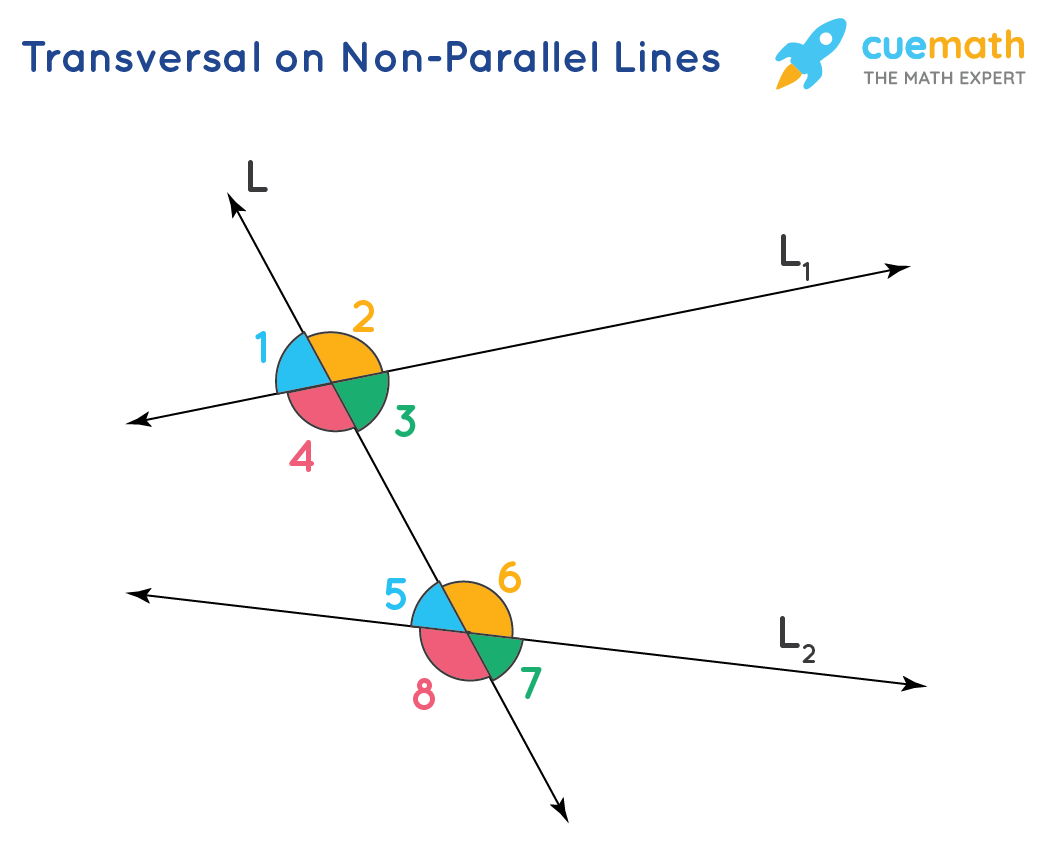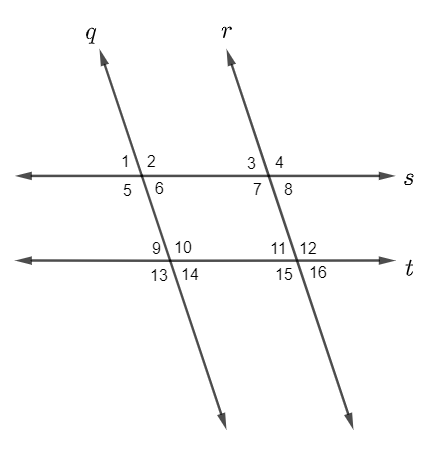Logic
Angle Names
Parallel and Perpendicular
Parallel Lines
Angle Measures
100
What does ^ mean?

and

100

two angles that are across from one another are called?

vertical angles

100

If 2 lines are parallel they have _______ slope.

same

100

What are parallel lines?

two lines that do not intersect and have the same slope

100

180 degrees

200

What is the inverse of the statement: If it is Thursday then I have geometry class.

If it is not thursday then I do not have geometry class.

200

What is the relationship between angle 2 and angle 8Alternate exterior

200

If I have the two equations:

y = 4x -6

y = -1/4x +10

What kind of lines are these?

perpendicular

200

What lines can i prove parallel if <1 = <9a and b

200

if angle 1 is 45 degrees what is the measure of angle 1445 degrees

300

What is the contrapositive of the statement: If it is raining, then I have an umbrella

If i do not have my umbrella, then it is not raining.

300

What is the relationship between angle 4 and angle 6?Alternate interior angles

300

Find the slope of the lines AC and BD

A(1, 0) C(-3, -2)

B(-4, -5) D(8, 7)

AC= 1/2

BD= 12/12 = 1

300

What lines can I prove parallel if <12 = <14M and N

300

If angle 7 is 100 degrees what is the measure of angle 11?80 degrees

400

Use the law of syllogism to make a conclusion:

If it is saturday, then I am at the movies.

If I am at the movies, then I get popcorn

If it is saturday, then I get popcorn

400

What is the relationship between angle 3 and angle 6?Consecutive interior angles

400

Write an equation for 2 parallel lines that have slopes of 2 and y intercepts at 0 and 4

y = 2x

y = 2x + 4

400

Why are A and B parallel if <3 + <6 = 180they are consecutive interior angles

400

If angle 2 is 3x + 7 and angle 10 is 2x + 20 what is the value of x13

500
Give an example of the law of detachment and a valid conclusion

***

500

What is the relationship between angle 5 and angle 2supplementary angles

500

A line has an equation of y = 3x + 2

Write an equation for a perpendicular line that passes through (3, 5)

y = -1/3x - 5

500

What lines are parallel if <10 = <12none because they are vertical angles

500

If angle 3 is 2x + 10 and angle 16 is 12x - 30

what is the measure of angle 318 degrees

Click to zoom